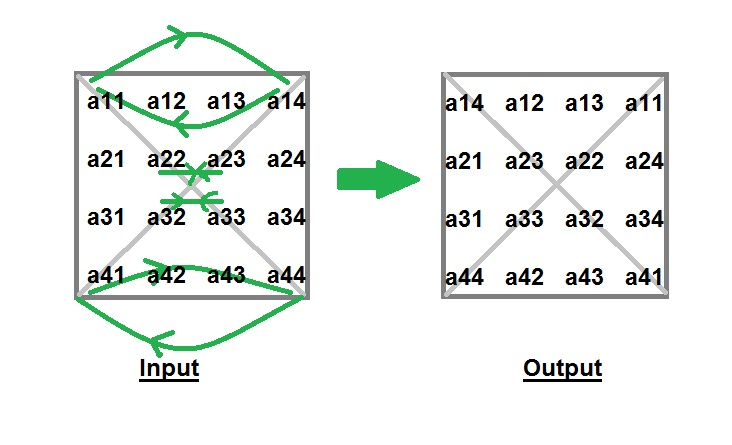# Javascript Program to Interchange Diagonals of Matrix

Given a square matrix of order n*n, you have to interchange the elements of both diagonals.
Examples :

```Input : matrix[][] = {1, 2, 3,
4, 5, 6,
7, 8, 9}
Output : matrix[][] = {3, 2, 1,
4, 5, 6,
9, 8, 7}

Input : matrix[][] = {4,  2,  3,  1,
5,  7,  6,  8,
9, 11, 10, 12,
16, 14, 15, 13}
Output : matrix[][] = {1,  2,  3,  4,
5,  6,  7,  8,
9, 10, 11, 12,
11, 14, 15, 16}```

Explanation : Idea behind interchanging diagonals of a square matrix is simple. Iterate from 0 to n-1 and for each iteration you have to swap a[i][i] and a[i][n-i-1].## Javascript

 ``

Output:

``` 6 5 4
1 2 3
9 8 7```

Time Complexity: O(N*N), as we are using nested loops for traversing the matrix.

Auxiliary Space: O(1), as we are not using any extra space.

Please refer complete article on Program to Interchange Diagonals of Matrix for more details!